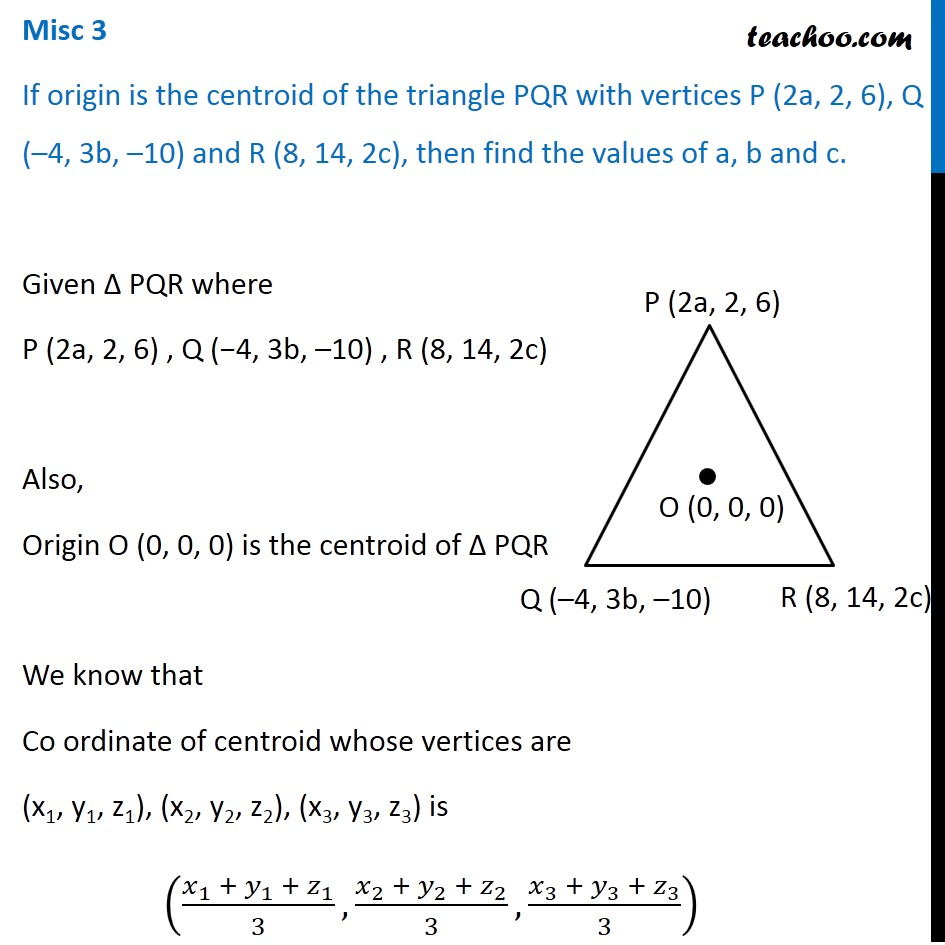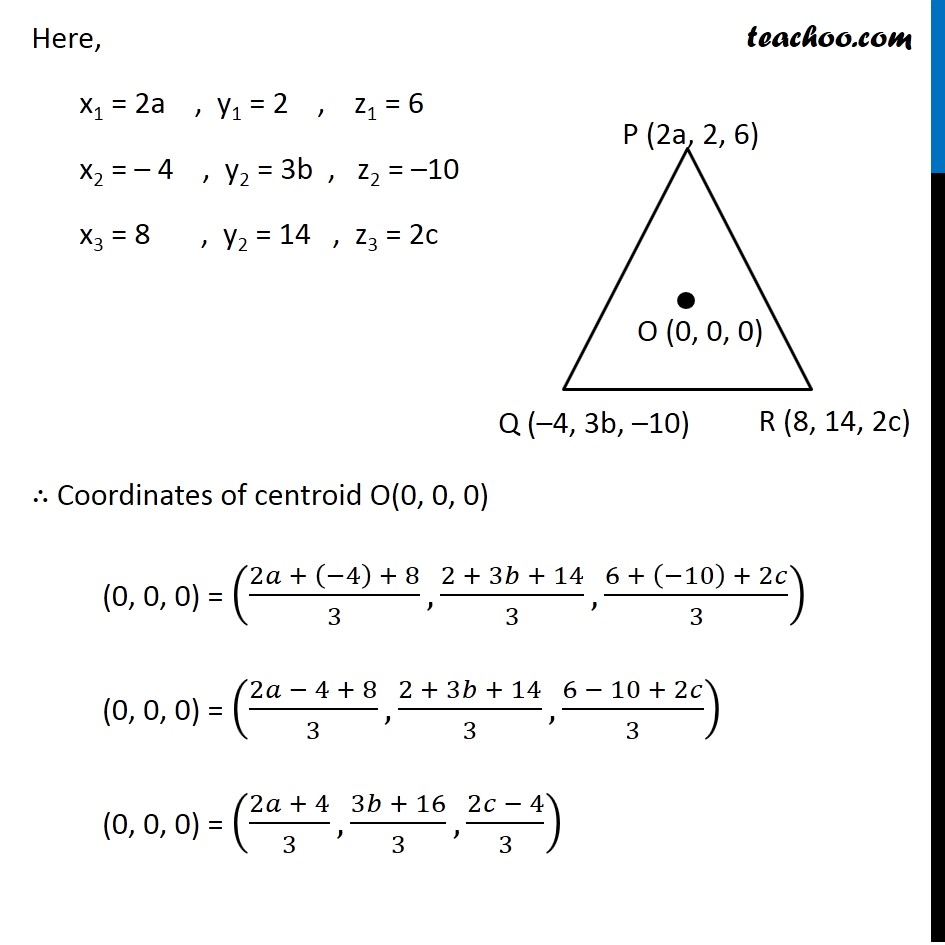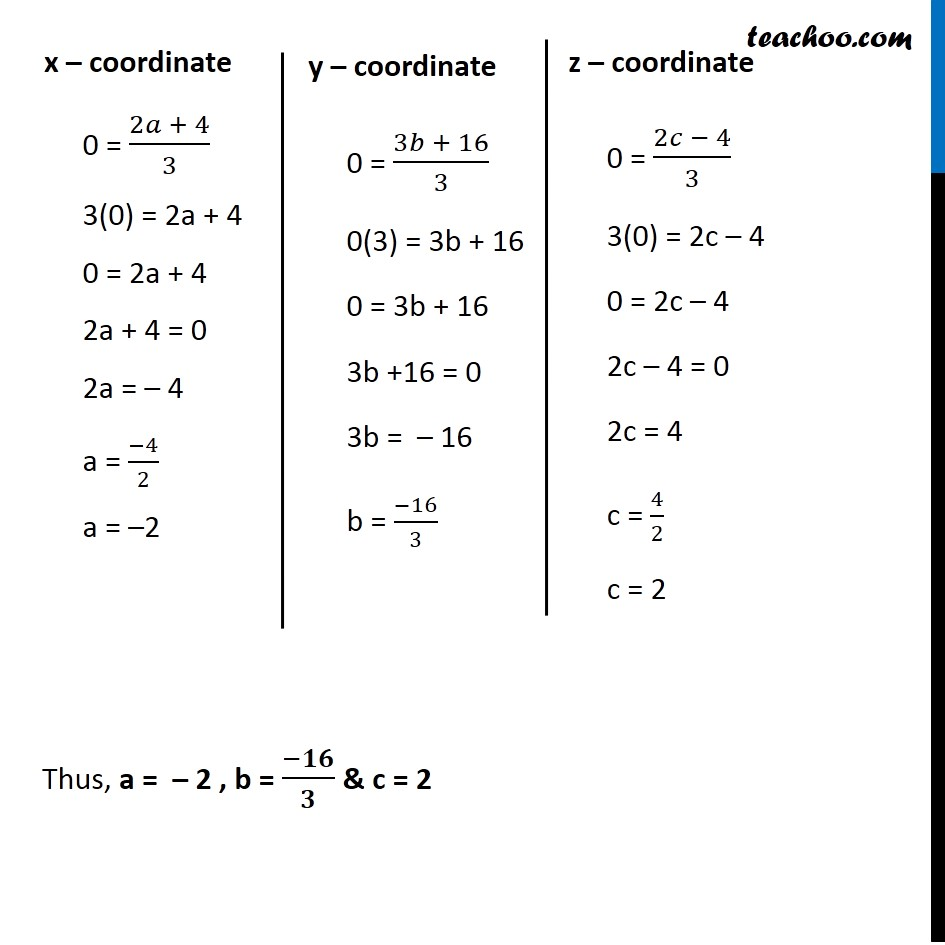Miscellaneous

Chapter 11 Class 11 - Intro to Three Dimensional Geometry
Serial order wiseLearn in your speed, with individual attention - Teachoo Maths 1-on-1 Class

### Transcript

Misc 3 If origin is the centroid of the triangle PQR with vertices P (2a, 2, 6), Q (–4, 3b, –10) and R (8, 14, 2c), then find the values of a, b and c. Given Δ PQR where P (2a, 2, 6) , Q (−4, 3b, –10) , R (8, 14, 2c) Also, Origin O (0, 0, 0) is the centroid of Δ PQR We know that Co ordinate of centroid whose vertices are (x1, y1, z1), (x2, y2, z2), (x3, y3, z3) is ((𝑥_1 + 𝑦_1 + 𝑧_1)/3,(𝑥_2 + 𝑦_2 + 𝑧_2)/3,(𝑥_3 + 𝑦_3 + 𝑧_3)/3) Here, x1 = 2a , y1 = 2 , z1 = 6 x2 = – 4 , y2 = 3b , z2 = –10 x3 = 8 , y2 = 14 , z3 = 2c ∴ Coordinates of centroid O(0, 0, 0) (0, 0, 0) = ((2𝑎 + (−4) + 8)/3,(2 + 3𝑏 + 14)/3,(6 + (−10) + 2𝑐)/3) (0, 0, 0) = ((2𝑎 − 4 + 8)/3,(2 + 3𝑏 + 14)/3,(6 − 10 + 2𝑐)/3) (0, 0, 0) = ((2𝑎 + 4)/3,(3𝑏 + 16)/3,(2𝑐 − 4)/3) x – coordinate 0 = (2𝑎 + 4)/3 3(0) = 2a + 4 0 = 2a + 4 2a + 4 = 0 2a = – 4 a = (−4)/2 a = –2 y – coordinate 0 = (3𝑏 + 16)/3 0(3) = 3b + 16 0 = 3b + 16 3b +16 = 0 3b = – 16 b = (−16)/3 z – coordinate 0 = (2𝑐 − 4)/3 3(0) = 2c – 4 0 = 2c – 4 2c – 4 = 0 2c = 4 c = 4/2 c = 2 Thus, a = – 2 , b = (−𝟏𝟔)/𝟑 & c = 2